Courses

# RD Sharma Solutions -Page No.9.20, Ratio Proportion And Unitary Method, Class 6, Maths Class 6 Notes | EduRev

## RD Sharma Solutions for Class 6 Mathematics

Created by: Abhishek Kapoor

## Class 6 : RD Sharma Solutions -Page No.9.20, Ratio Proportion And Unitary Method, Class 6, Maths Class 6 Notes | EduRev

The document RD Sharma Solutions -Page No.9.20, Ratio Proportion And Unitary Method, Class 6, Maths Class 6 Notes | EduRev is a part of the Class 6 Course RD Sharma Solutions for Class 6 Mathematics.
All you need of Class 6 at this link: Class 6

#### PAGE NO 9.20:

Question 1: If a = 2b, then a : b =

(a) 2 : 1
(b) 1 : 2
(c) 3 : 4
(d) 4 : 3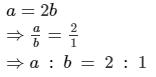Hence, the correct option is (a).

Question 2: If 4 : 3 = x2 : 12, then the value of x (x > 0).

(a) 16
(b) 4
(c) 9
(d) 3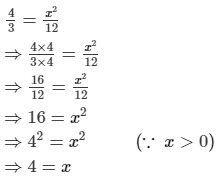Hence, the correct option is (b).

Question 3: If x : y = 2 : 3 and y : z = 2 : 3, then x : z =

(a) 2 : 3
(b) 3 : 4
(c) 5 : 7
(d) 4 : 9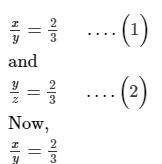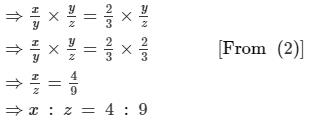Hence, the correct option is (d).

Question 4: If 80 : 60 = x : 12, then x =

(a) 16
(b) 7
(c) 24
(d) 50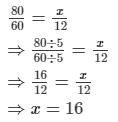Hence, the correct option is (a).

Question 5: Two numbers are in the ratio 3 : 5 and their sum is 96. The larger number is

(a) 36
(b) 42
(c) 60
(d) 70

ANSWER: Let the larger number be x.
Then, the smaller number be (96 − x).
According to the question,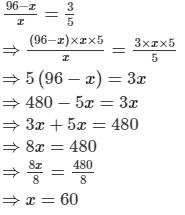Thus, the larger number is 60.
Hence, the correct option is (c).

#### Question 6: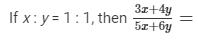(a) 7/11
(b) 17/11
(c) 17/23
(d) 4/5

It is given that,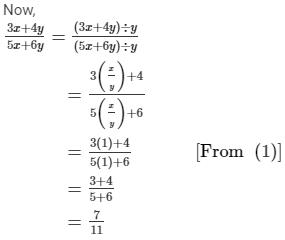Hence, the correct option is (a).

Question 7: If six men can do a piece of work in 6 days, then 3 men can do same work in

(a) 10 days

(b) 12 days

(c) 15 days

(d) 18 days

ANSWER: Let the required number of days be x.

Number of days is inversaly proportional to the number of men.

According to the question,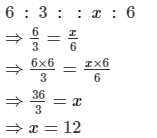Thus, 3 men can do same work in 12 days.
Hence, the correct option is (b).

Question 8:If 4 : 5 : : x : 45, then x =

(a) 54

(b) 60

(c) 36

(d) 30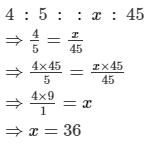Hence, the correct option is (c).

Question 9: If 343 is the third proportional of a and b, where a : b = 1 : 7, then the value of a + b is

(a) 14

(b) 24

(c) 56

(d) 63

a/b = 1/7        ...(1)

According to the question,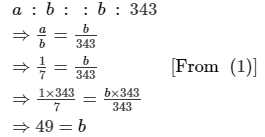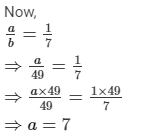Thus, a + b = 7 + 49 = 56
Hence, the correct option is (c).

Question 10: The first three terms of a proportion are 12, 21 and 8 respectively. Then 4th term is
(a) 18
(b) 16
(c) 14
(d) 20

ANSWER: Let the 4th term be x.
According to the question,
12 : 21 : : 8 : x
⇒Product of extreme terms = product of mean terms
⇒12x =8×21
12x=16812x12=16812x=1

⇒12x  = 168

⇒12x/2 =168/2

⇒ x = 14

Hence, the correct option is (c).

Question 15: If a, b, c, are in proportion, then

(a) a2 = bc

(b) b2 = ac

(c) c2 = ab

(d) None of these

∵ a , b and c are in proportion.

∴ a : b :: b : c

⇒ a/b = b/c

⇒ b2 = ac

Question 16:If the cost of 5 bars of a soap is Rs. 30, then the cost of one dozen bars is

(a) Rs 60

(b) Rs 120

(c) Rs 72

(d) Rs 14

#### ANSWER:(c) Rs. 72Let the cost of one dozen bars be Rs. x.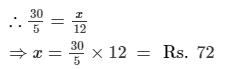Cost of one dozen (12) bars = Rs. 72

Question 17: 12 men can finish a piece of work in 25 days. The number of days in which the same piece of work can be done by 20 men, is
(a) 10 days
(b) 12 days
(c) 15 days
(d) 14 days

It is given that 12 men can finish a piece of work in 25 days.
Let the number of days required to do the same piece of work by 20 men be days.
We get: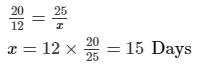Question 18: If the cost of 25 packets of 12 pencils each is Rs 750, then the cost of 30 packets of 8 pencils each is

It is given that the cost of 25 packets of 12 pencils each is Rs 750; therefore, we have:

Cost of (25×12) pencils = 300 pencils = Rs. 750

Let the cost of (30×8) or 240 pencils be Rs. x.

∵ 750 : 300 : : x : 240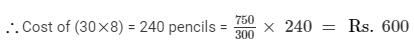Question 19: If a, b, c are in proportion, then
(a) a : b : : b : c
(b) a : b : : c : a
(c) a : b : : c : b
(d) a : c : : b : c

ANSWER: (a) a : b :: b : c
⇒ ac = b2

Question 20: The first, second and fourth terms of a proportion are 16, 24 and 54 respectively. The third term is
(a) 32
(b) 48
(c) 28
(d) 36

The first, second and fourth term of a proportion are 16, 24 and 54, respectively.
Let third term is be x.
According to the question, we have:
16 : 24 = x : 54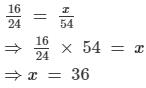Offer running on EduRev: Apply code STAYHOME200 to get INR 200 off on our premium plan EduRev Infinity!

103 docs

,

,

,

,

,

,

,

,

,

,

,

,

,

,

,

,

,

,

,

,

,

,

,

,

,

,

,

,

,

,

;